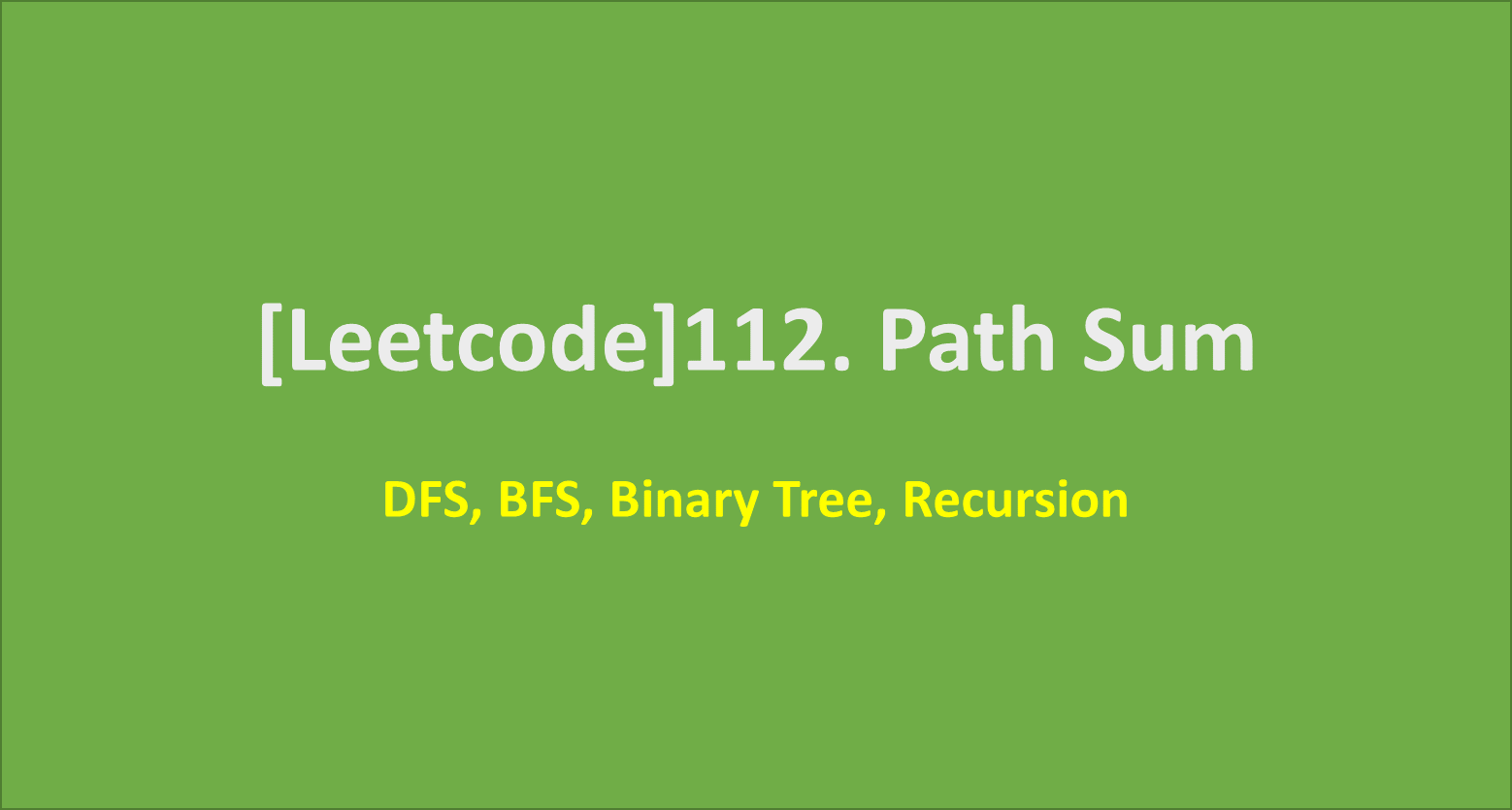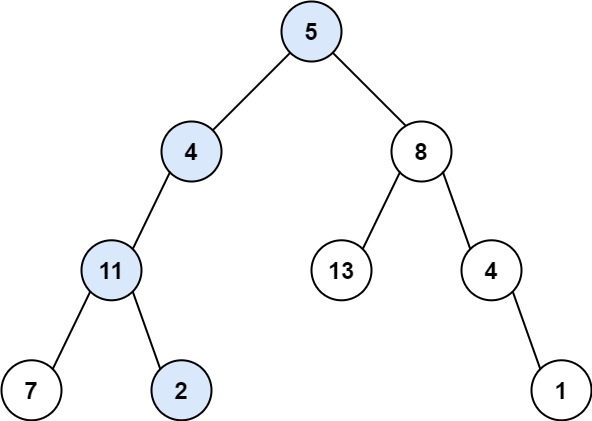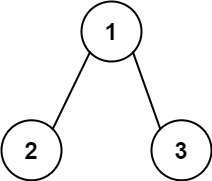# [Leetcode]112. Path Sum

limelimejiwon·2022년 3월 22일
0

## Python으로 공부하는 코딩테스트

목록 보기
21/67## 📄 Description

Given the root of a binary tree and an integer targetSum, return true if the tree has a root-to-leaf path such that adding up all the values along the path equals targetSum.

A leaf is a node with no children.

### Example 1:Output: true
Explanation: The root-to-leaf path with the target sum is shown.

### Example 2:Output: false
Explanation: There two root-to-leaf paths in the tree:
(1 --> 2): The sum is 3.
(1 --> 3): The sum is 4.
There is no root-to-leaf path with sum = 5.

### Example 3:

Input: root = [], targetSum = 0
Output: false
Explanation: Since the tree is empty, there are no root-to-leaf paths.

## 🔨 My Solution

• DFS using stack

### 🔵 Rules

• stack contains elements of (current node, sum)
• pop(0) ensures the DFS approach, that it will go down the tree until it reaches leaf node.
• if the curr_node is a leaf node,
=> If the node_sum is same as targetSum, return True
=> If not, it will continue with the next node.
• If the while loop stops, it means there is no PathSum, so return False.

## 💻 My Submission

class Solution:
def hasPathSum(self, root: Optional[TreeNode], targetSum: int) -> bool:

# if the tree is empty, there are no roof-to-leaf path
if root is None:
return False

stack=[(root, 0)]

# DFS using stack
while stack:
cur_node,node_sum=stack.pop()
node_sum+=cur_node.val
# if the node is leaf node
if cur_node.left is None and cur_node.right is None:
if node_sum==targetSum: return True
if cur_node.left: stack.append((cur_node.left,node_sum))
if cur_node.right: stack.append((cur_node.right,node_sum))
return False

## Other solutions (1) DFS Recursively

class Solution:
def hasPathSum(self, root: Optional[TreeNode], targetSum: int) -> bool:
# DFS recursively
if root is None:
return False

res=[]
self.recursion(root, targetSum, res)
return any(res)

def recursion(self, cur_node, target, res):
if cur_node.left is None and cur_node.right is None:
if cur_node.val==target: res.append(True)
if cur_node.left: self.recursion(cur_node.left, target-cur_node.val, res)
if cur_node.right: self.recursion(cur_node.right, target-cur_node.val, res)


### 💡 What I learned

1. any() function
• This function receives iterable object(list, string, dictionary etc.)
• If at least one element of an iterable is true, it returns True.
• If all elements are false or if an iterable is empty it returns False.

## Improved version of DFS Recursively

def hasPathSum1(self, root, sum):
if not root:
return False
if not root.left and not root.right and root.val == sum:
return True
return self.hasPathSum(root.left, sum-root.val) or self.hasPathSum(root.right, sum-root.val)

## Other solutions (2) BFS using queue

class Solution:
def hasPathSum(self, root: Optional[TreeNode], targetSum: int) -> bool:
# BFS using queue
if root is None:
return False

queue=[(root, 0)]

while queue:
node,node_sum=queue.pop(0)
node_sum+=node.val
if not node.left and not node.right:
if node_sum==targetSum: return True
if node.left: queue.append((node.left, node_sum))
if node.right: queue.append((node.right, node_sum))
return False